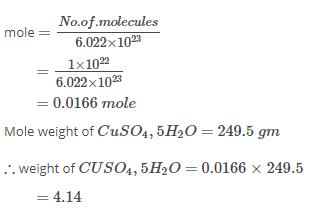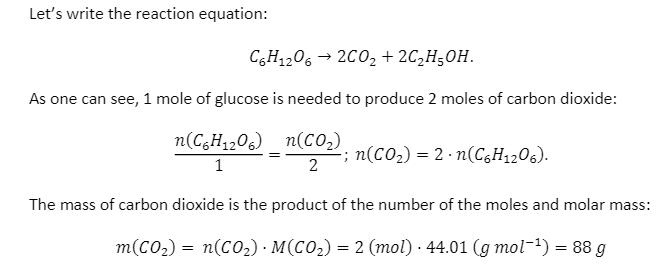Courses

# Test: Mass & Stoichiometry

## 15 Questions MCQ Test Chemistry Class 11 | Test: Mass & Stoichiometry

Description
This mock test of Test: Mass & Stoichiometry for JEE helps you for every JEE entrance exam. This contains 15 Multiple Choice Questions for JEE Test: Mass & Stoichiometry (mcq) to study with solutions a complete question bank. The solved questions answers in this Test: Mass & Stoichiometry quiz give you a good mix of easy questions and tough questions. JEE students definitely take this Test: Mass & Stoichiometry exercise for a better result in the exam. You can find other Test: Mass & Stoichiometry extra questions, long questions & short questions for JEE on EduRev as well by searching above.
QUESTION: 1

### For the reaction 2NaN3(s) → 2Na(s) + 3N2 (g), how many moles of sodium azide (NaN3) must react in order to produce 8.75 moles of nitrogen gas?​

Solution:

2NaN3 → 2Na + 3N2
As above reaction is already balanced we can write:
2 moles of NaN3 ≡ 3 moles of N2
So, moles of NaN3 ≡ 8.75 moles of N2
8.75 x 2/3 = 5.83 moles of NaN3

QUESTION: 2

### Amount of water produced by the combustion of 16g of methane is:

Solution:

C H₄ + 2O₂  => CO₂ + 2 H₂O  complete combustion with out CO.

1 mole of methane  16 grams reacts with 2 moles of oxygen = 64 grams . to produce 2 moles of water = 36 grams.

So 16 grams of methane produces 36 grams of water.

QUESTION: 3

### Branch of chemistry in which quantities of reactants and products of a chemical reaction are determined is known as:

Solution:

Stoichiometry comes from the Greek “stoiechion” (element) and “metron” (to measure). As such, stoichiometry deals with determining the amounts of reactants and products that are consumed and produced within a given chemical reaction.

The stoichiometric coefficient of any species that does not participate in a given chemical reaction is zero.

The principles of stoichiometry are based upon the law of conservation of mass. Matter can neither be created nor destroyed, so the mass of every element present in the product(s) of a chemical reaction must be equal to the mass of each and every element present in the reactant(s).

QUESTION: 4

The mass of 1 Χ 1022 molecules of CuSO4.5H2O is:​

Solution:QUESTION: 5

The mass of one mole of NaOH will be (At. mass of Na = 23, O = 16, H = 1)​

Solution:

Mass of 1 mole of NaOH = 23 + 16 + 1 = 40

QUESTION: 6

If 2 moles of H2 and O2 are available for the reaction, identify the limiting reagent for the reaction,
2H2 + O2 → 2H2O​

Solution:

As we know that the limiting reagent is the substance that is totally consumed in reaction. So when we take 2 moles of H2 and O2 then the H2 molecules are totally consumed in the reaction. So H2 is the limiting reagent.

QUESTION: 7

Which of the following has more number of atoms?

Solution:

Neon's atomicity is 1 Ozone's atomicity is 3 Oxygen's atomicity is 2 Carbon monoxide's atomicity is 2 Now Ozone has more atom in a molecule than any other gas in the options. So in 1 mole or 6.022×1023 Ozone has more atom.

QUESTION: 8

Molecular mass of glucose molecule (C6H12O6) is

Solution:

Mass of C=12g
Mass of H =1g
Mass of O =16g
Thus,
Mass of C6H12O6= 12×6+1×12+16×6
=72+12+96
=180g

QUESTION: 9

If 0.5 mole of BaCl2 is mixed with 0.2 mole of Na3PO4 ,then maximum number of moles of Ba3(PO4)2 that can be formed​

Solution:

Balanced equation for reaction between BaCl2 and Na3PO4 is as follows:

3BaCl2 + 2Na3PO4 → Ba3(PO4)2  + 6NaCl

3 moles of BaCl2 react with 2 moles of Na3PO4 to give 1 mole of Ba3(PO4)2

0.5 moles of BaCl2 will react with (2/3) x 0.5 = 0.33 moles of Na3PO4

Available moles of Na3PO4 = 0.2

So, Na3PO4 is the limiting reagent

Now, 2 moles of Na3PO4 give 1 mole of Ba3(PO4)2

So, 0.2 moles of Na3PO4 will give ½ x 0.2 = 0.1 mole of Ba3(PO4)2

Hence, maximum number of moles of Ba3(PO4)2 formed = 0.1

QUESTION: 10

The atomic mass of an element is usually fractional because:

Solution:

The atomic masses of most elements are fractional because they exist of a mixture of Isotopes of different masses. the fractional atomic masses arise because of this mixture.
Mass =total mass of all atoms/number of atom.

QUESTION: 11

If you have 2 moles of A and 3 moles of B, Identify the limiting reagent in the following reaction.

A + B → AB​

Solution:

A + B → AB divide given moles of each reactant by their stoichiometric coefficient , the one with least ratio is limit reagents divide with their co efficient A=2/1 =2 B=3/1 =3. So A is the limiting reagent.

QUESTION: 12

According to the chemical equation,

Mg(s) + 2HCl → MgCl2(aq) + H2(g)

How many moles of magnesium are needed to produce 12.34 moles of hydrogen gas?​

Solution:

1mole of Mg gives 1mole water so, to produce 12.34 moles of water we required 12.34mole of Mg.

QUESTION: 13

Two different atoms A and B combine together to form AB. What will be AB?

Solution:

A molecule is an electrically neutral group of two or more atoms held together by chemical bonds. Molecules are distinguished from ions by their lack of electrical charge.

QUESTION: 14

20 L of H2 gas at S.T.P weigh about:​

Solution:

1 mole of hydrogen (about 2 g) occupies a volume of 22.4 L at S.T.P
Hence, weight of 20 L of H2 at S.T.P = (2 x 20)/22.4 = 1.8g

QUESTION: 15

One type of anaerobic respiration converts glucose, C6H12O6, to ethanol C2​H5​OH and carbon dioxide. If the molecular weight of glucose is 180 grams/mol and the molar mass of ethanol is 50 g/mol how many grams of carbon dioxide are produced when 1 mol of glucose is digested via respiration?

Solution: